# ISEE Lower Level Quantitative : How to find the whole from the part

## Example Questions

### Example Question #1 : How To Find The Whole From The Part

Jonathan ate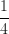of a carton of ice cream.  This is equal to 1 pound of ice cream.  How many pounds of ice cream were in the carton of ice cream before Jonathan started eating?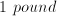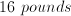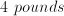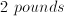Explanation:

The fractionmeans that Jonathan has eaten 1 part out of a possible 4 parts of the carton of ice cream.  So we can multiply the amount that he ate (1 pound) by 4 to see that there are 4 pounds of ice cream in a carton.

### Example Question #2 : How To Find The Whole From The Part

37 is 20% of what number?

185

7.4

74

135

185

Explanation:

To find the whole from the part, divide the “part” by the percent. In order to do this, first change the percent into a decimal (20% becomes 0.2).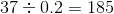Therefore, 37 is 20% of 185.

### Example Question #3 : How To Find The Whole From The Part

What is 3.4 rounded to the nearest whole number?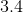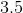Explanation:

A whole number is one in which the one's place is the smallest unit. Therefore, in a whole number, there is no decimal. While any number that ends in a .5 decimal or greater rounds up, anything that is smaller than .5 rounds down. Therefore,would round down to.

### Example Question #4 : How To Find The Whole From The Part

Brad is buying two appliances from a store: a microwave that costs $30 and a toaster that costs$25. He has two coupons: 10 percent off 1 item, and $5 off one item. What is the most money that he can save with these coupons (in dollars)? Possible Answers: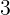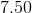Correct answer:Explanation: If Brad has 2 coupons, 10 percent off 1 item, and$5 off one item, he should use the 10 percent off coupon on the more expensive item to save more money.

Since the $30 microwave is more expensive, he should use the 10 percent off coupon on this item. This will save him$3 because 10 percent of $30 is$3.

He can then use the $5 off coupon on the$25 toaster, which saves him $5. Therefore, the total savings is$8.

### Example Question #5 : How To Find The Whole From The Part

A classroom contains 30 students, and there are the same number of boys as girls. Ifof the boys in the classroom are wearing sandals , how many boys are wearing sandals?Explanation:

If the classroom of 30 students has an equal number of boys and girls, then there are 15 girls and 15 boys.

Ifof the boys are wearing sandals, then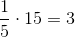boys are wearing sandals.

### Example Question #6 : How To Find The Whole From The Part

One fourth of the bananas that Beth bought are not ripe. The rest are ripe. She has 9 ripe bananas. How many bananas are there in total?Explanation:

If one fourth of the bananas that Beth bought are not ripe, then three fourths are ripe. Therefore, the total number of bananas can be represented byin the equation below: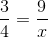First, we cross multiply: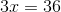Next, we divide by 3: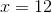Therefore, there are a total of 12 bananas.

### Example Question #7 : How To Find The Whole From The Part

Amanda and Adriana each ran four laps around the track in twelve minutes. At the same rate of speed, how long would they need to run twenty-eight laps?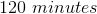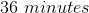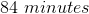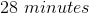Explanation:

To solve this problem, one must first determine how long it takes to run one lap. Since it takes twelve minutes to run four laps, divide twelve by four.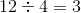It takes three minutes to run one lap.

To determine how long it will take to run twenty-eight laps, multiply the original rate of speed by the new distance.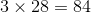It will take eighty-four minutes to run twenty-eight laps if each lap is run in three minutes.